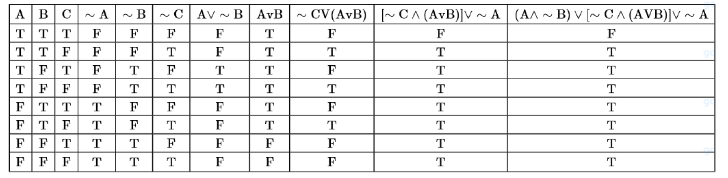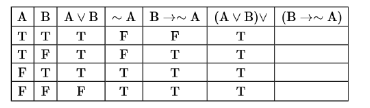# Let F1 (A,B,C) =Question:

Let $F_{1}(A, B, C)=(A \wedge \sim B) \vee[\sim C \wedge(A \vee B)] \vee \sim A$ and $F_{2}(A, B)=(A \vee B) \vee(B \rightarrow \sim A)$ be two logical expressions.

Then:

1. (1) $\mathrm{F}_{1}$ is not a tautology but $\mathrm{F}_{2}$ is a tautology

2. (2) $\mathrm{F}_{1}$ is a tautology but $\mathrm{F}_{2}$ is not a tautology

3. (3) $\mathrm{F}_{1}$ and $\mathrm{F}_{2}$ both area tautologies

4. (4) Both $\mathrm{F}_{1}$ and $\mathrm{F}_{2}$ are not tautologies

Correct Option: 1

Solution:

Truth table for $\mathrm{F}_{1}$Not a tautology

Truth table for $\mathrm{F}_{2}$$\mathrm{F}_{1}$ not shows tautology and $\mathrm{F}_{2}$ shows tautology